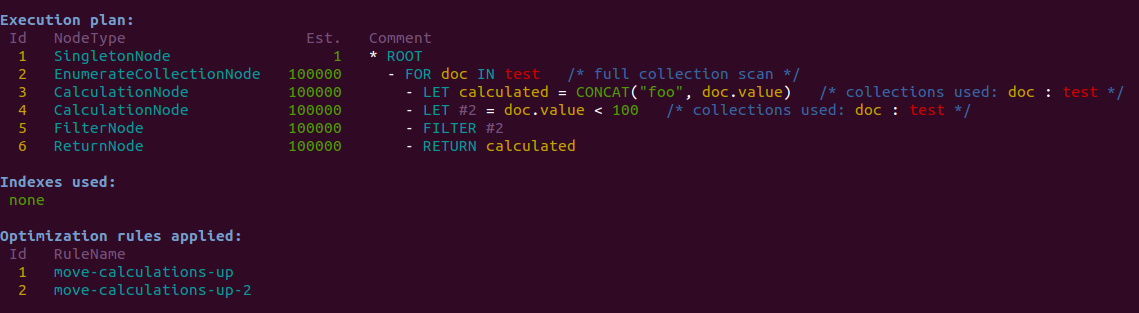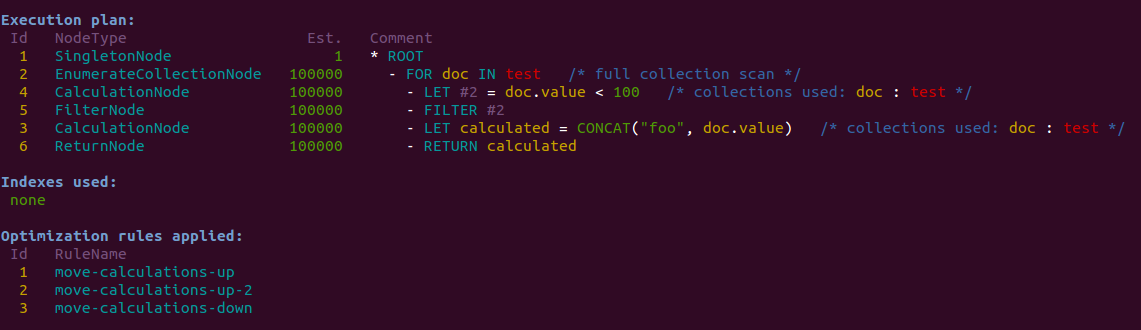# YAOR - Yet Another Optimizer Rule

A quick post that showcases the new optimizer rule `move-calculations-down` that was added in ArangoDB 2.5 (current `devel` branch).

Consider the following simple query:

If no indexes are present in collection `test`, the execution plan will look like this in 2.4:The plan can be improved a bit. While there are no indexes to exploit, the calculation for `calculated` can be pushed beyond the execution of the `FILTER`. This will be very beneficial if the calculation is expensive and the `FILTER` can prune a lot of documents.

In 2.5, there is an optimizer rule `move-calculations-down` that will do this. It will move all eligible calculations as far down in the plan as possible. A calculation obviously can be moved down a step only if the successor step does not depend on its result.

Additionally, a calculation will not be moved beyond a `COLLECT` operation, because `COLLECT` changes which variables are visible in a scope. Finally, a calculation will not be moved down inside a `FOR` loop, in order to avoid repeated calculations.

In the example query, the step following the `LET` calculation was a `FILTER`. The filter condition does not depend on the result of `calcuated`, so the calculation is eligible for being moved down.

The resulting execution plan will look like this in 2.5:As we can see from the changed id sequence on the left, the calculation was moved down.

What does this buy us? For the simple query above, with the `test` collection containing 100.000 documents with `value` ranging from 0 to 99,999, the results are as follows:

• if the filter leaves only 0.1 % of the documents pass, execution time goes down from 0.87 seconds to 0.17 seconds thanks to the rule
• if the filter lets 1 % of the documents pass, execution time is 0.21 seconds with the rule, and 0.91 without
• if the filter lets 10 % of the documents pass, execution time is 0.25 s, vs. 0.91 seconds without.
• if the filter lets all documents pss, there is no difference in execution time

This is quite a nice speedup, especially when taking into account how simple the optimizer rule is. The effects may be even greater for queries that contain multiple calculations that can be pushed beyond filters, or for more expensive calculations.

Of course the best solution for the above query would be to use a skiplist index on `value`, but that’s a different story. The optimizer rule shown here is orthogonal to using indexes, so queries already using indexes might still benefit from the rule if they contain additional calculations or further filters which cannot be satifisfied by indexes.